Courses

# JEE Main Physics Mock - 3

## 25 Questions MCQ Test Mock Test Series for JEE Main & Advanced 2022 | JEE Main Physics Mock - 3

Description
This mock test of JEE Main Physics Mock - 3 for JEE helps you for every JEE entrance exam. This contains 25 Multiple Choice Questions for JEE JEE Main Physics Mock - 3 (mcq) to study with solutions a complete question bank. The solved questions answers in this JEE Main Physics Mock - 3 quiz give you a good mix of easy questions and tough questions. JEE students definitely take this JEE Main Physics Mock - 3 exercise for a better result in the exam. You can find other JEE Main Physics Mock - 3 extra questions, long questions & short questions for JEE on EduRev as well by searching above.
QUESTION: 1

Solution:
QUESTION: 2

### The de-Broglie wavelength associated with proton changes by 0.25% if its momentum is changed by P₀. The initial momentum was

Solution: Use the formula lamba=h/p you will see that they are inversely related, so if you reduce wavelength by 0.25% then momentum reduces by 1 unit. So that implies Initial momentum is 401Po
QUESTION: 3

### A fixed volume of iron is drawn into a wire of length l. The extension x produced in this wire by a constant force F is proportional to

Solution: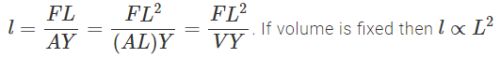QUESTION: 4

Condensers of capacities 2 μ F and 3 μ F are connected in series and a condenser of capacity 1 μ F is connected in parallel with them. The resultant capacity is

Solution:
QUESTION: 5

Vapour is injected at a uniform rate in a closed vessel which was initially evacuated. The pressure in the vessel

Solution: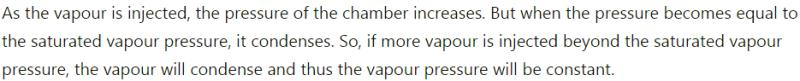QUESTION: 6

What is the coefficient of mutual inductance, when the magnetic flux changes by 2X10-2 Wb and change in current is 0.01 A ?

Solution:
QUESTION: 7

The electric potential V is given as a function of distance x (metre) by V = (5x2 + 10x - 9) volt. Value of electric field at x = 1m is

Solution:

V = 5x2 + 10x - 9
E = - dv dx
= - (10x + 10)
= -(10 x 1 + 10)
= - 20 V/m

QUESTION: 8

If 3.8 x 10-6 is added to 4.2 x 10-5 giving due regard to significant figures, then the result will be

Solution: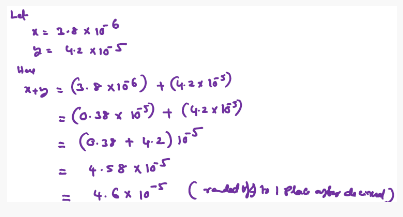QUESTION: 9

The mass and diameter of a planet have twice the value of the corresponding parameters of earth. Acceleration due to gravity on the surface of the planet is

Solution:
QUESTION: 10

Referring to the above two questions, the acceleration due to gravity is given by

Solution:
QUESTION: 11
In the following question, a Statement of Assertion (A) is given followed by a corresponding Reason (R) just below it. Read the Statements carefully and mark the correct answer-
Assertion(A): Pendulum of a clock is made of alloys and not of pure metals.
Reason(R): Use of alloys make the pendulum look good.
Solution:
QUESTION: 12
Among electron, proton, neutron and $\alpha$ - particle the maximum penetration capacity is for
Solution:
QUESTION: 13
In the following question, a Statement of Assertion (A) is given followed by a corresponding Reason (R) just below it. Read the Statements carefully and mark the correct answer-
Assertion(A): Lifting of aircraft is caused by pressure difference brought by varying speed of air molecules.
Reason(R): As the wings/aerofoils move against the wind, the streamlines crowd more above them than below, causing higher velocity above than below.
Solution:
QUESTION: 14
The spectrum of light from an incandescent source is
Solution: The spectrum of colors produced by incandescent lights is continuous, more similar to that which is produced by the sun, and incandescent lights transmit more red wavelengths than fluorescent light.
QUESTION: 15

The ratio of densities of nitrogen and oxygen is 14 : 16. The temperature at which the speed of sound in nitrogen will be same as that of oxygen at 55 oC is

Solution: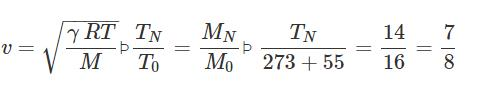p p TN=287K=14∘C
QUESTION: 16

The wavelength of ultrasonic waves in air is of the order of

Solution:
QUESTION: 17
A bullet hits and gets embedded in a solid block resting on frictionless surface. In this process which one of the following is correct?
Solution:
QUESTION: 18

Two rods of the same length and diameter having thermal conductivities K1 and K2 are joined in parallel. The equivalent thermal conductivity of the combination is

Solution:
QUESTION: 19
A wave front travels
Solution:
QUESTION: 20

The Figure shows a rectangular loop of resistance R, width l and length a being pulled at a constant speed vthrough a region of thickness d(>a)d>a in which a uniform magnetic field of induction BB acting into the plane of paper is set up. The position of the loop at instant t is indicated by x, the distance penetrated by the right edge of the loop into the field with x = 0 at t = 0. (see diagram). Ignore the fringe effects on the magnetic field on the loop.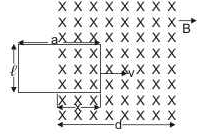Q. If a graph is plotted for the flux φB through the loop as a function of the coil position x, which of the following will show the correct graph?

Solution:
*Answer can only contain numeric values
QUESTION: 21

If a radioactive material is decayed by 29.28% in 10 minute, then its half life will be:-

Solution: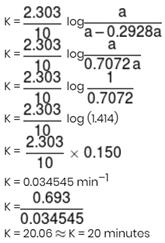*Answer can only contain numeric values
QUESTION: 22

The elevator shown in figure is descending with an acceleration of 2 ms–2. The mass of the block A = 0.5 kg. The force exerted by the block A on the block B is 2x (take g = 10 ms–2). find the value of x.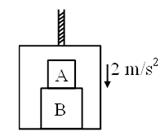Solution:

Let A applies force N1 on B
Then B also applies an opposite force N1 on A
As shown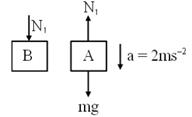For A    mg – N1 = ma
N1 = m(g – a) = 0.5 (10 – 2)
N1 = 4
N1 = 2x ⇒ x = 2

*Answer can only contain numeric values
QUESTION: 23

A Wheatstone's bridge is balanced with a resistance of 625 Ω in the third arm,  where P, Q and S are in the 1st, 2nd and 4th arm respectively. If P and Q are interchanged, the resistance in the third arm has to be increased by 51Ω to secure balance. The unknown resistance in the fourth arm is 6.5 × 10N Ω, then N will be :-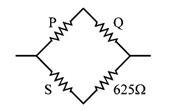Solution: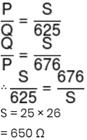*Answer can only contain numeric values
QUESTION: 24

If an objects is placed symmetrically between two plane mirrors, inclined at an angle of 72°, then the total number of images formed is:-

Solution:
*Answer can only contain numeric values
QUESTION: 25

Two tuning forks A and B vibrating simultaneously produce 5 beats. Frequency of B is 512. It is seen that if one arm of A is filed, then the number of beats increases. Frequency of A will be:-

Solution:

After filling frequency increases, so nincreases(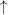­). Also it is given that beat frequency increases (i.e., x­)
Hence nA­– nB = x­   ...(i)               → Correct
nB – nA­= x­...(ii)             → Wrong
⇒ nA = nB + x = 512 + 5 = 517 Hz.